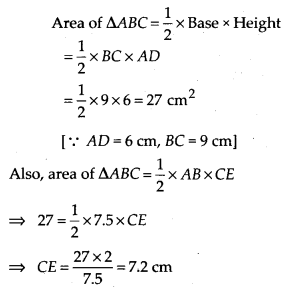## MP Board Class 7th Maths Solutions Chapter 11 Perimeter and Area Ex 11.2

Question 1.
Find the area of each of the following parallelograms: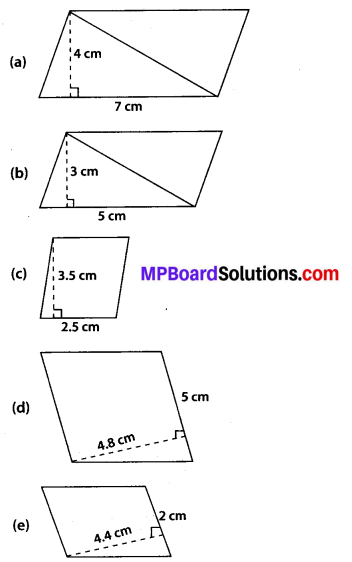Solution:
Area of parallelogram = Base × Height
(a) Height = 4 cm, Base = 7 cm
Area of parallelogram = 7 × 4 = 28 cm2

(b) Height = 3 cm, Base = 5 cm
Area of parallelogram = 5 × 3 = 15 cm2

(c) Height = 3.5 cm, Base = 2.5 cm
Area of parallelogram = 2.5 × 3.5 = 8.75 cm2

(d) Height = 4.8 cm, Base = 5 cm
Area of parallelogram = 5 × 4.8 = 24 cm2

(e) Height = 4.4 cm, Base = 2 cm
Area of parallelogram = 2 × 4.4 = 8.8 cm2

Question 2.
Find the area of each of the following triangles: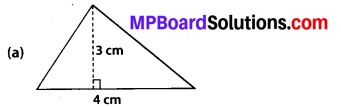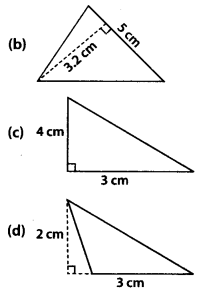Solution:
Area of triangle = $$\frac{1}{2}$$ × Base × Height
(a) Base = 4 cm, height = 3 cm
Area = $$\frac{1}{2}$$ × 4 × 3 = 6 cm2

(b) Base = 5 cm, height = 3.2 cm
Area = $$\frac{1}{2}$$ × 5 × 3.2 = 8 cm2

(c) Base = 3 cm, height = 4 cm
Area = $$\frac{1}{2}$$ × 3 × 4 = 6cm2

(d) Base = 3 cm, height = 2 cm
Area = $$\frac{1}{2}$$ × 3 × 2 = 3 cm2Question 3.
Find the missing values: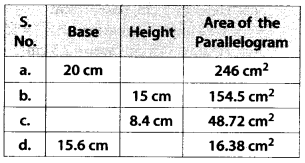Solution:
Area of parallelogram = Base × Height
(a) Base = 20 cm
Let height = h
Area of parallelogram = 246 cm2
∴ 20 × h = 246
⇒ h = $$\frac{246}{20}$$ = 12.3 cm
Therefore, the height of parallelogram is 12.3 cm.

(b) Let base = b
Height = 15 cm
Area of parallelogram = 154.5 cm2
∴ b × 15 = 154.5
⇒ b = $$\frac{154.5}{15}$$ = 10.3 cm
Therefore, the base of parallelogram is 10.3 cm.

(c) Let base = b
Height = 8.4 cm
Area of parallelogram = 48.72 cm2
∴ b × 8.4 = 48.72
⇒ b = $$\frac{48.72}{8.4}$$ = 5.8 cm
Therefore, the base of parallelogram is 5.8 cm.

(d) Base = 15.6 cm
Let height = h
Area of parallelogram = 16.38 cm2
∴15.6 × h = 16.38
⇒ h = $$\frac{16.38}{15.6}$$ = 1.05 cm
Therefore, the height of parallelogram is 1.05 cm.

Question 4.
Find the missing values: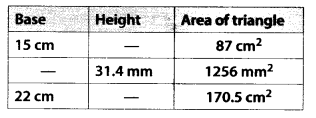Solution:
Area of triangle = $$\frac{1}{2}$$ × Base × Height
Let b be the base of triangle and h be the height of triangle.
(i) b = 15 cm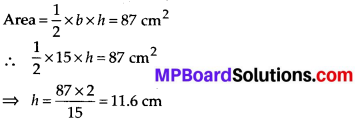Therefore, the height of triangle is 11.6 cm.

(ii) h = 31.4 mm
Area = $$\frac{1}{2}$$ × b × h = 1256 mm2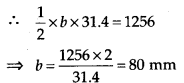Therefore, the base of triangle is 80 mm.

(iii) b = 22 cm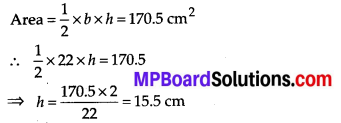Therefore, the height of triangle is 15.5 cm.

Question 5.
PQRS is a parallelogram (see the given figure). QM is the height from Q to SR and QN is the height from Q to PS. If SR = 12 cm and QM = 7.6 cm. Find:
(a) the area of the parallelogram PQRS
(b) QN, if PS = 8 cm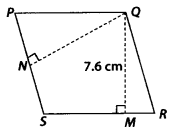Solution:
(a) Area of parallelogram = Base × Height
= SR × QM
= 12 × 7.6 = 91.2 cm2

(b) PS = 8 cm
Area of parallelogram = Base × Height
= PS × QN = 91.2 cm2
⇒ 8 × QN = 91.2
⇒ QN = $$\frac{91.2}{8}$$ = 11.4 cm

Question 6.
DL and BM are the heights on sides AB and AD respectively of parallelogram ABCD (see the given figure).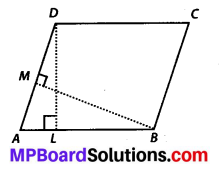If the area of the parallelogram is 1470 cm2, AB = 35 cm and AD = 49 cm, find the length of BM and DL.
Solution:
Area of parallelogram = Base × Height
= AB × DL
⇒ 1470 = 35 × DL
⇒ DL = $$\frac{1470}{35}$$ = 42 cm
Also, area of parallelogram = AD × BM
⇒ 1470 = 49 × BM
∴ BM = $$\frac{1470}{49}$$ = 30 cmQuestion 7.
∆ABC is right angled at A (see the given figure). AD is perpendicular to BC. If AB = 5 cm, BC – 13 cm and AC = 12 cm, find the area of ∆ABC. Also find the length of AD.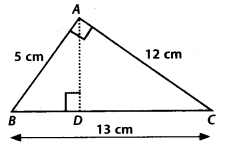Solution: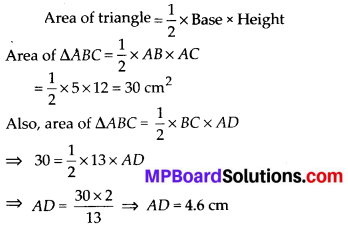Question 8.
∆ABC is isosceles with AB = AC= 7.5 cm and BC = 9 cm (see the given figure). The height AD from A to BC, is 6 cm. Find the area of ∆ABC. What will be the height from C to AB i. e., CE?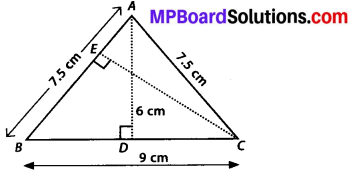Solution: